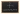# Coordinate Planes: Practicing Segments

POSTED ON MAY 15, 2020## Becoming Familiar with the Coordinate Plane

Coordinate planes are important to understand because they help us know how to read graphs, understand points in space, and even apply concepts in other subjects like data science and coding!

Read Genesis' Intro to Coordinate Planes lesson first to understand what coordinate planes are and how to use them. Then, use what you've learned with the segments in coordinate planes __practice questions__ on this page to practice finding colinearity and midpoints of segments.

Once you've checked your answers below, you can also keep practicing with Genesis' Warmup Problems for Coordinate Planes.

## Practicing Segments

Master segments in coordinate planes with these 10 practice problems! If you need help or get stuck, find the answers and Genesis' video tutorial below.

1. Draw a coordinate plane and label the x and y axes. The x-axis runs from -5 to 5, and the y-axis runs from -5 to 5.
2. Draw a segment AB such that point A has coordinates (3,4) and point B has coordinates (1,4).
3. Draw a segment FG such that point F has coordinates (-5, 2) and point G has coordinates (-5, -2).
4. Draw a segment AB such that point A has coordinates (4, -1), point B has coordinates (4, -5), and there is a midpoint C at coordinates (4, -3).
5. Draw a segment FG such that point F has coordinates (3, 2), point G has coordinates (9, 2), and there is a midpoint H at coordinates (6, 2).
6. Is the point (-4, 6) on segment KL?
7. Is the point (-2,-1) on segment ON?
8. Is point S a midpoint of segment QR?
9. Is point V a midpoint of segment TU?
10. Challenge yourself: Draw a segment AB with coordinates of your choice such that it has a midpoint C. What is the length of your segment? Where is the midpoint on your segment?

## Solution Walkthrough

1.2.3.4.5.6. Yes

7. No

8. Yes

9. No

10. The segment drawn for this problem depends on the student. An example of an acceptable answer to this problem is a segment 4 units in length with a midpoint that divides the segment into two units.

## More Practice for Coordinate Planes

We hope you enjoyed Genesis' Drill Problems for Segments! Continue practicing coordinate planes with the warmup problems below. Or, review key terms and concepts with Genesis' Intro to Coordinate Planes lesson and video.

## Keep Learning

We hope you enjoyed Genesis' Lesson on Points, Lines, and Coordinate Planes! This lesson falls under our Pre-Algebra B course curriculum.

To keep practicing or learning, please check out all of our math tutorials on our Math Practice page.

## Need Help?

Looking up your questions is one of the best ways to learn! Another great way to learn is from an experienced math instructor. Learn more about our group and private math classes with live instructors, or our math curriculum.